# OHM'S LAW

Click or Tap the Example circuits below to invoke TINACloud and select the Interactive DC mode to Analyze them Online.
Get a low cost access to TINACloud to edit the examples or create your own circuitsTHREE PHASE NETWORKS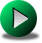SERIES CONNECTED RESISTORS

The magnitude of current flowing in a circuit is dependent upon both voltage and resistance. The relationship among these three electrical properties (current, voltage and resistance) is the important and well-known Ohm's law, which states that the current flow in a circuit is directly proportional to the applied source voltage and inversely proportional to the resistance of the circuit.

In mathematical form:or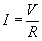In the following examples, we generally give three solutions for each problem.

• Numerical solution by TINA
• Solution by TINA's Interpreter, using Ohm's law
• Solution by formulas using Ohm's law

#### Example 1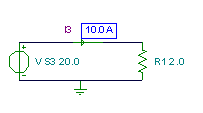Click/tap the circuit above to analyze on-line or click this link to Save under Windows

Using Ohm's law: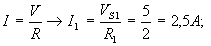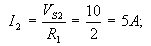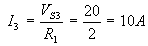The calculated currents confirm that current is directly proportional to the source voltage.

{Using TINA's Interpreter}
I1:=VS1/R1;
I1=[2.5]
I2:=VS2/R1;
I2=
I3:=VS3/R1;
I3=

#### Example 2

In the following example, you can verify that the current is inversely proportional to the resistance.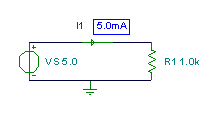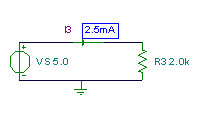Click/tap the circuit above to analyze on-line or click this link to Save under Windows

Using Ohm's law: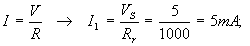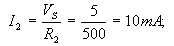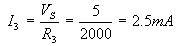{Using TINA's Interpreter}
I1:=VS/R1;
I1=[5m]
I2:=VS/R2;
I2=[10m]
I3:=VS/R3;
I3=[2.5m]

#### Example 3

In this example, you can see that the voltage across a resistor is directly proportional to the value of its resistance.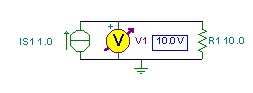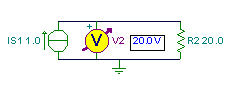Click/tap the circuit above to analyze on-line or click this link to Save under Windows

While the detailed formula isn't shown, it is used in TINA's Interpreter to evaluate the example.
{Using TINA's Interpreter}
V1:=IS1*R1;
V1=
V2:=IS1*R2;
V2=
V3:=IS1*R3;
V3=

#### Example 4

In this example, you can check that the voltage across a resistor is directly proportional to the current flowing through the resistor and to the resistor's resistance.

Click/tap the circuit above to analyze on-line or click this link to Save under Windows

While the detailed formula isn't shown, it is used in TINA's Interpreter to evaluate the example.
{Using TINA's Interpreter}
V1:=IS1*R1;
V1=
V2:=IS2*R1;
V2=
V3:=IS3*R1;
V3=THREE PHASE NETWORKSSERIES CONNECTED RESISTORS